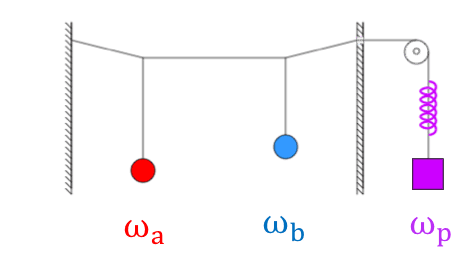# Coupled pendulum with external force?

questionnerI got this picture from a superconducting parametric amplifier text I was reading.
(The picture is a mechanical analog of a non-degenerate parametric amplifier.)

If the balls(red and blue) were oscillating at their own natural frequencies, and an external force is driven(purple), how would the balls' frequencies be determined?
If the problem is too sophisticated, then assuming that wp = wa + wb, then would the balls just get amplified with wa and wb conserved?

(Actually the second question is what I am interestedLast edited by a moderator:
•osilmag

Gold Member
The period, and thus the frequency of a pendulum, depends upon length and gravity (##T=2pi \sqrt{L/g}##). So, I would state that ##dT/dt## would be proportional to ##dL/dt##, the length being the parameter the force is changing.

Gold Member
I worked it out that it could be ##dT/dL = (2pi^2)/(Tg)##.

Gold Member
Then your force would change the length ##dL/dt=-k*dy/dt##.

Last edited:
Homework Helper
@questionner: the picture you show is of three coupled oscillators, not two with an independent external force !

questionner
The period, and thus the frequency of a pendulum, depends upon length and gravity (##T=2pi \sqrt{L/g}##). So, I would state that ##dT/dt## would be proportional to ##dL/dt##, the length being the parameter the force is changing.
Okay thank you for the reply. So is the answer yes? or no?

questionner
@questionner: the picture you show is of three coupled oscillators, not two with an independent external force !
Actually the right most one is not a pendulum. Sorry for the misunderstanding, but it's a two coupled oscillator!

Gold Member
I got this picture from a superconducting parametric amplifier text I was reading.

Does it contain a differential equation that describes the behavior of the amplifier?

(The picture is a mechanical analog of a non-degenerate parametric amplifier.)

Then there should be an analogous differential equation that describes its motion.

Gold Member
Okay thank you for the reply. So is the answer yes? or no?

You asked how the pendulum frequencies would be changed and I put forward a possible way to find how they're changing. I was speculating how the contraption works since I have limited info on the driver. To me it looks like a driven spring.

Last edited:
zoki85
Summary:: Here's an interesting situation(picture): when the two pendulums(red and blue) oscillate and there is an external force(purple), how would their frequencies change?

View attachment 254134
I got this picture from a superconducting parametric amplifier text I was reading.
(The picture is a mechanical analog of a non-degenerate parametric amplifier.)

If the balls(red and blue) were oscillating at their own natural frequencies, and an external force is driven(purple), how would the balls' frequencies be determined?
If the problem is too sophisticated, then assuming that wp = wa + wb, then would the balls just get amplified with wa and wb conserved?

(Actually the second question is what I am interestedNo. Motion of harmonically driven 2 coupled oscillators is more complicated. There are generally two resonant frequencies of the system ω',ω" depending on ωab and coupling (the expressions are not very short/nice ). If ωp=ω' or ωp=ω" than the amplification condition occurs